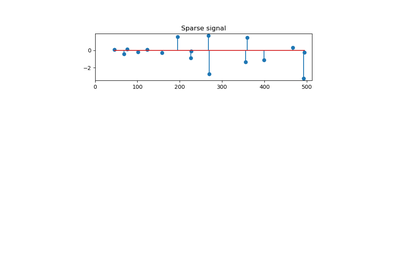# `sklearn.datasets`.make_sparse_coded_signal¶

`sklearn.datasets.``make_sparse_coded_signal`(n_samples, n_components, n_features, n_nonzero_coefs, random_state=None)[source]

Generate a signal as a sparse combination of dictionary elements.

Returns a matrix Y = DX, such as D is (n_features, n_components), X is (n_components, n_samples) and each column of X has exactly n_nonzero_coefs non-zero elements.

Read more in the User Guide.

Parameters: n_samples : int number of samples to generate n_components : int, number of components in the dictionary n_features : int number of features of the dataset to generate n_nonzero_coefs : int number of active (non-zero) coefficients in each sample random_state : int, RandomState instance or None, optional (default=None) If int, random_state is the seed used by the random number generator; If RandomState instance, random_state is the random number generator; If None, the random number generator is the RandomState instance used by np.random. data : array of shape [n_features, n_samples] The encoded signal (Y). dictionary : array of shape [n_features, n_components] The dictionary with normalized components (D). code : array of shape [n_components, n_samples] The sparse code such that each column of this matrix has exactly n_nonzero_coefs non-zero items (X).

## Examples using `sklearn.datasets.make_sparse_coded_signal`¶Orthogonal Matching Pursuit